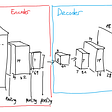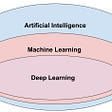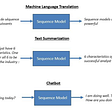# Custom Implementation of Performance Metrics from Scratch

Why??

we have an Sklearn library in python to compute various performance matrics from data. we can use that library and can compute F1 score,accuracy score ,AUC score etc..

then, why custom implementation from scratch..

simple…to understand the math behind it…Its always welcome when you know the basic mathematics behind some libraries..it will be helpful to us when we are working on complex problems and there we need to create some algorithm from our own when all others failed…

Performance Metrics

There are many performance metrics by which we can evaluate our models performance,they are F1 score,AUC score,accuracy score,confusion metrics etc..

In this blog we are going to compute following performance metrics from scratch..

1. Confusion Metrics
2. F1 Score
3. AUC score
4. Accuracy Score
5. Mean Square Error (MSE)
6. MAPE
7. R² Error

Lets Begin Coding….!!!

import data first…

1. Confusion Metrics

confusion matrix consists of matrix of elements namely True Positive,False Positive,False Negative,True Negative

2.F1 Score

F1-score means a statistical measure of the accuracy of a test or an individual. It is composed of two primary attributes, viz. precision and recall, both calculated as percentages and combined as harmonic mean to assign a single number, easy for comprehension.
Recall=True positive/(True positive+False Negative)
Precision=True positive/(True positive+False positive)
F1 is usually more useful than accuracy, especially if you have an uneven class distribution.

3.AUC Score

The area under the ROC curve, or AUC, is used as a measure of classifier performance
AUC=0.5 is the accuracy of making classification decisions

4.Accuracy Score

Accuracy score basically means ratio of number of points correctly classified to Total number of Points.
which means, Accuracy Score=(TP+TN)/(TP+TN+FP+FN)

5.Mean Square Error(mse)

The mean squared error tells you how close a regression line is to a set of points. It does this by taking the distances from the points to the regression line (these distances are the “errors”) and squaring them. The squaring is necessary to remove any negative signs.

6.MAPE

The mean absolute percentage error (MAPE) is the mean or average of the absolute percentage errors of forecasts.

The formulae for MAPE is =Summation(((Actual_val-Predicted_val)/Actual_val)*100)/Total no of points in data
But,What if Actual_val==0,than summation will gets false ,To avoid this problem we took average of all actual points and replace Actual_val in denometer of MAPE formulae by this average

7.R² Error

R-squared is a statistical measure of how close the data are to the fitted regression line. It is also known as the coefficient of determination, or the coefficient of multiple determination for multiple regression.

The definition of R-squared is fairly straight-forward; it is the percentage of the response variable variation that is explained by a linear model. Or:

R-squared = Explained variation / Total variation

You can visit my github profile for further details regarding the same blog..The Link is:

https://github.com/akshayashokbhor/performance-metrics-from-scratch-

Blog By:

Akshay Bhor: Deep learning Engineer

an Data Scientist

--

--

--

## More from Akshay Bhor ..an Data Scientist

Love podcasts or audiobooks? Learn on the go with our new app.## Semi-supervised learning with autoencoders## A Dive into Deep Learning- Part 1## Word Level English to Bengali Machine Translation Using Encoder-Decoder Model